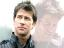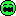# Engrenages/gears

2 replies to this topic

### #1Tchernoben

Tchernoben

Casio Fan

•• Members
•• 42 posts
• Location:Nice
• Interests:Calculators, Pc, mangas, warhammer.

• Calculators:
Ti 89 Titanium

Posted 05 April 2006 - 06:47 PM

Hello everybody

Sorry for not being too much present here...
for apologize I want to show you an idea I had about the similarities between Coroutines in lua and gears.
then, on the base of circle, I have programmed a physical model which can recreate the gear movement, like a clock in fact ( I think I'll need objects in the further version).
The code above is only the gear creating function, please try it and tell me what you think about that idea.
```require("draw")
showgraph(1)
draw.onbuffer(1)
pi = 3.141592654

function rouage ( x,y,r,t,pas,o,p,a )
e = 0
for i=t, 2*pi+pas , pas do
e = e+1
if e%2 == 0 then
r = r+a
draw.line ( x+(r-a)*math.cos(i), y+(r-a)*math.sin(i), x+r*math.cos(i), y+r*math.sin(i) )
else
r = r-a
draw.line ( x+(r+a)*math.cos(i), y+(r+a)*math.sin(i), x+r*math.cos(i), y+r*math.sin(i) )
end
draw.line ( x+r*math.cos(i), y+r*math.sin(i), x+r*math.cos(i+pas), y+r*math.sin(i+pas) )
r = r - pas*o
end
end

roue1 = coroutine.create (
function ()
pa = pi/5
while true do
for j=0, 2*pa, 0.03*pi do
rouage ( 75,100,50,j,pa,0,1,10)
coroutine.yield ()
end
end
end )

while not testkey(K_EXE) do
draw.clear ()
coroutine.resume ( roue1 )
draw.update ()
end```

Hope you'll enjoy It

See ya later

### #2Major

Major

Newbie

•• Members
•• 17 posts
• Location:Toulouse, France.

• Calculators:

Posted 06 April 2006 - 08:27 PM

D?sol? Tchernoben c'?tait une erreur ! Tu peux donc supprimer ce message ainsi que le pr?c?dent. Merci.### #3Tchernoben

Tchernoben

Casio Fan

•• Members
•• 42 posts
• Location:Nice
• Interests:Calculators, Pc, mangas, warhammer.

• Calculators:
Ti 89 Titanium

Posted 07 April 2006 - 05:30 PM

Ok c'est fait

I am trully sorry but this program I began 3 days ago will be interuptedbecause Lua is too slow (maybe am I too bad on it) for the kind of program I wanted to realize...
I think I'll do it with C on the SDK#### 0 user(s) are reading this topic

0 members, 0 guests, 0 anonymous users